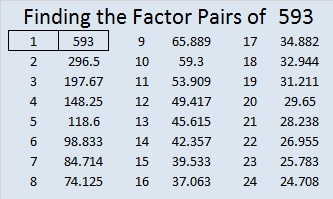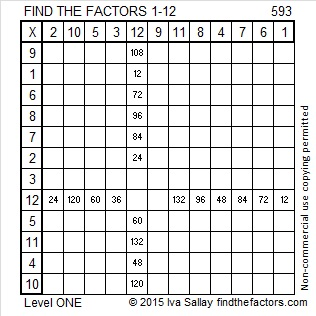# 593 and Level 1

593 is the sum of the seven prime numbers from 71 to 101 as well as the nine prime numbers from 47 to 83.

When the number formed from the last two digits of prime number 593 is divided by 4, the remainder is 1 so 593 can be expressed at the sum of two squares AND is the hypotenuse of a primitive Pythagorean triple. Specifically:

23² + 8² = 593

593 is the hypotenuse of the primitive Pythagorean triple prime 368-465-593Print the puzzles or type the solution on this excel file: 12 Factors 2015-08-24

—————————————————————————————————

• 593 is a prime number.
• Prime factorization: 593 is prime.
• The exponent of prime number 593 is 1. Adding 1 to that exponent we get (1 + 1) = 2. Therefore 593 has exactly 2 factors.
• Factors of 593: 1, 593
• Factor pairs: 593 = 1 x 593
• 593 has no square factors that allow its square root to be simplified. √593 ≈ 24.35159How do we know that 593 is a prime number? If 593 were not a prime number, then it would be divisible by at least one prime number less than or equal to √593 ≈ 24.4. Since 593 cannot be divided evenly by 2, 3, 5, 7, 11, 13, 17, 19, or 23, we know that 593 is a prime number.

—————————————————————————————————